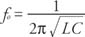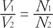## Resonance

From the general expression for Ohm's law of the ac circuit, the current can be writtenThe equation indicates that the current has its maximum value when the impedance has its minimum value. The frequency for this situation is called the resonant frequency. It is defined as## Transformers

A transformer is an ac version of the induction coil applied to transform voltages from one circuit to another. The device is constructed of two coils with differing number of turns wrapped on a common iron core. In Figure , assume that is the output (secondary) voltage.Figure 1 A simple transformer.

The ratio of input to output voltage iswhere N 1 and N 2 are the respective number of turns of wire of each coil. Depending upon the number of turns in each coil, the transformer can increase (step up) or decrease (step down) the voltage. This equation is for an ideal transformer. Actual transformers have efficiencies of 90 to 99 percent.